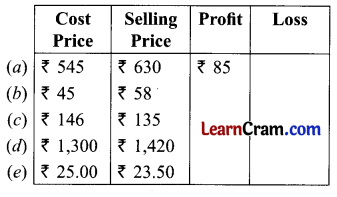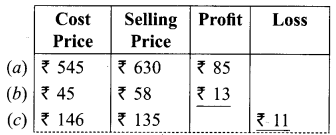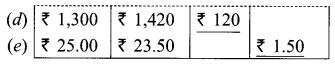# DAV Class 5 Maths Chapter 11 Worksheet 1 Solutions

The DAV Books Solutions Class 5 Maths and DAV Class 5 Maths Chapter 11 Worksheet 1 Solutions of Profit and Loss offer comprehensive answers to textbook questions.

## DAV Class 5 Maths Ch 11 Worksheet 1 Solutions

Question 1.
Complete the following table. The first one is done for you.Solution:
If S.P. > C.P. then Profit.
If C.P. > S.P. then loss.Question 2.
Solve the following questions:
(a) If the cost price of a book is ₹ 50.50 and the selling price is ₹ 65, find the profit or loss.
Solution:
C.P. of book = ₹ 50.50
S.P. of book = ₹ 65.00
As S.P. > C.P. we have a profit
Profit = ₹ 65.00 – ₹ 50.50 = ₹ 14.50

(b) If the cost price of a doll is ₹ 195 and the selling price is ₹ 181.50, find the profit or loss.
Solution:
C.P. of doll = ₹ 195
S.P. of doll = ₹ 181.50
As C.P. > S.P. we have a loss
Loss = ₹ 195.00 – ₹ 181.50 = ₹ 13.50

(c) The cost price of a pen is ₹ 19 and the selling price is ₹ 24. Is there a gain or loss? How much?
Solution:
C.P. of pen = ₹ 19
S.P. of pen = ₹ 24
As S.P. > C.P. we have a profit
Profit = ₹ 24 – ₹ 19 = ₹ 5(d) The cost price of a washing machine is ₹ 12,450. If the selling price is ₹ 12,075 what is the gain or loss?
Solution:
C.P. of washing machine = ₹ 12,450
S.P. of washing machine = ₹ 12,075
As C.P. > S.P. we have a loss
Loss = ₹ 12,450 – ₹ 12,075 = ₹ 375

DAV Class 5 Maths Chapter 11 Worksheet 1 Notes

Cost Price:
The rate at which an article is purchased is called cost price (C.P).

Selling Price:
The rate at which an article is sold is called selling price (S.P.).

e.g., An article like Walkman purchased from the market at the rate ₹ 800 and sold at ₹ 1000.
The purchasing rate is the cost price and the selling rate is called the selling price of the article.

When the selling price (S.P.) is greater than the cost price (C.P), We have a Gain (profit)
S.P. > C.P. → Gain

When the cost price (C.P.) is greater than the selling price (S.P.), We have a loss.
C.P. > S.P. → Loss

Selling Price = Cost Price + Profit
Selling Price = Cost Price – Losse.g. Sashi purchases a T-shirt for ₹ 175. He sold it for ₹ 200. Find whether Sashi has profit or loss.
Solution:
C.P. = ₹ 175; S.P. = ₹ 200
As S.P. > C.P. Sashi has a profit.
Sashi has profit of ₹ 25 (200 – 175 = ₹ 25)

e.g. Raju purchases a pencil box for ₹ 35. There were some defects in the box. So he sold it for ₹ 30. Find the got profit or loss.
Solution:
Cost Price = ₹ 35
Selling Price = ₹ 30
As C.P. > S.P.
so he has loss of ₹ 5 (₹ 35 – ₹ 30 = ₹ 5).